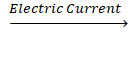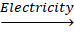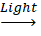Class X - Science

# Chapter - 1 Chemical Reactions and Equations NCERT Solution

Other Chapters

Page 6

Q1. Why should a Magnesium ribbon be cleaned before burning in air?

Solution: When left unused, the outer layer of Magnesium metal reacts with the atmospheric oxygen and forms Magnesium Oxide (MgO) layer which is a very stable compound thus preventing further reactions with Oxygen. It is therefore necessary to clean the ribbon by brushing it to remove the layer of MgO so that the reaction becomes feasible with the atmospheric oxygen.

Q2. Write the balanced equations for the following chemical reactions.

i) Hydrogen + Chlorine→ Hydrogen Chloride

ii) Barium Chloride + Aluminium Sulphate Barium Sulphate + Aluminium Chloride

iii) Sodium + Water → Sodium Hydroxide + Hydrogen

Solution:

i) H2 + Cl2 2HCl

ii) 3BaCl2 + Al2(SO4)3 2AlCl3 + 3BaSO4

iii) 2Na + 2H2O 2NaOH + H2

Q3. Write a balanced chemical equation with state symbols for the following reactions.

i) Solutions of Barium Chloride and Sodium Sulphate in water react to give insoluble Barium Sulphate and solution of Sodium Chloride.

ii) Sodium Hydroxide solution (in water) reacts with Hydrochloric Acid solution (in water) to produce Sodium Chloride solution and water.

Solution:

i) BaCl2 + Na2SO4BaSO4 + 2NaCl

ii) NaOH + HCl NaCl + H2O

Page 10

Q1. A solution of a substance ‘X’ is used for white washing.

i) Name the substance ‘X’ and write its formula.

ii) Write the reaction of the substance ‘X’ named in (i) above with water.

Solution:

(i) The substance ‘X’ which is used in whitewashing is quick lime or Calcium Oxide and its formula is CaO.

(ii) CaO + H2O Ca(OH)2

Q2. Why is the amount of gas collected in one of the test tubes in Activity 1.7 double of the amount collected in the other? Name this gas.

Solution:

The chemical reaction involved in the electrolysis of water is

2H2O(l)2H2(g) + O2(g)

Water (H2O) contains two parts hydrogen and one part oxygen. Therefore, the amount of hydrogen and oxygen produced during electrolysis of water is in a 2:1 ratio. During electrolysis, since hydrogen goes to one test tube and oxygen goes to another, the amount of gas collected in one of the test tubes is double of the amount collected in the other.

Page 13

Q1. Why does the colour of Copper Sulphate solution change when an iron nail is dipped in it?

Solution:

When an iron nail is dipped in the copper sulphate solution, the iron displaces copper from the copper sulphate since iron is more reactive than copper. The colour of the copper sulphate solution changes due to this displacement. The reaction is -

Fe + CuSO4 FeSO4 + Cu

Q2. Give an example of a double displacement reaction other than the one given in Activity 1.10.

Solution:

Reactions in which there is an exchange of ions between the reactants are called double displacement reactions.

2KBr(aq) + BaI2(aq) → 2KI(aq) + BaBr2(aq)

Q3. Identify the substances that are oxidized and the substances that are reduced in the following reactions.

(i) 4Na(s) + O2(g) → 2Na2O(s)

(ii) CuO(s) + H2(g) → Cu(s) + H2O(l)

Solution:

(i) Sodium (Na) is oxidized with the addition of Oxygen (O2)

(ii) Copper (Cu) is reduced when Hydrogen (H2) is added to CuO.

Exercise

Q1. Which of the statements about the reaction below are incorrect?

2PbO(s) + C(s) → 2Pb(s) + CO2(g)

(b) Carbon Dioxide is getting oxidised

(c) Carbon is getting oxidised

(d) Lead Oxide is getting reduced

(i) (a) and (b)

(ii) (a) and (c)

(iii) (a), (b) and (c)

(iv) all

Solution: (i) (a) and (b)

Explanation: (a) because Oxygen is being removed and (b) because the removed oxygen from Lead is added to the elemental Carbon.

Q2. Fe2O3 + 2Al Al2O3 + 2Fe

The above reaction is an example of a

(a) Combination reaction

(b) Double displacement reaction.

(c) Decomposition reaction.

(d) Displacement reaction.

Solution: (d) Displacement reaction.

Explanation: The Oxygen from the Ferrous Oxide is getting displaced to the Aluminium metal to form Aluminium Oxide.

Q3. What happens when dilute hydrochloric acid is added to iron fillings? Tick the correct answer.

(a) Hydrogen gas and Iron chloride are produced.

(b) Chlorine gas and Iron hydroxide are produced.

(c) No reaction takes place.

(d) Iron salt and water are produced.

Solution: (a) Hydrogen gas and Iron Chloride are produced.

Explanation: The Chlorine from the Hydrogen Chloride is displaced due to addition of the Iron fillings undergoing the following reaction.

2HCl + Fe FeCl2 + H2

Q4. What is a balanced chemical equation? Why should a chemical equation be balanced?

Solution:

A balanced chemical equation is the one in which the number of atoms on both the sides of the chemical equation (the reactant side and the product side) of a reaction are equal. The chemical equation needs to be balanced so that it obeys the Law Of Conservation of Mass.

Q5. Translate the following statements into chemical equations and balance them.

(a) Hydrogen gas combines with nitrogen to form ammonia.

(b) Hydrogen Sulphide gas burns in air to give water and Sulphur Dioxide.

(c) Barium Chloride reacts with Aluminium Sulphate to give Aluminium Chloride and a precipitate of Barium Sulphate.

(d) Potassium metal reacts with water to give Potassium Hydroxide and Hydrogen gas.

Solution:

(a) Unbalanced: H2 + N2 NH3

Balanced: 3H2 + N2 2NH3

(b) Unbalanced: H2S + O2 H2O + SO2

Balanced: 2H2S + 3O2 2H2O + 2SO2

(c) Unbalanced: BaCl2 + Al2(SO4)3 AlCl3 + BaSO4

Balanced: 3BaCl2 + Al2(SO4)3 2AlCl3 + 3BaSO4

(d) Unbalanced: K + H2O KOH + H2

Balanced: 2K + 2H2O 2KOH + H2

Q6. Balance the following chemical equations.

(a) HNO3 + Ca(OH)2 Ca(NO3)2 + H2O

(b) NaOH + H2SO4 Na2SO4 + H2O

(c) NaCl + AgNO3 AgCl + NaNO3

(d) BaCl2 + H2SO4 BaSO4 + HCl

Solution:

(a) 2HNO3 + 2Ca(OH)2 2Ca(NO3)2 + 2H2O

(b) 6NaOH + 3H2SO4 H2SO4 + 6H2O

(c) NaCl + AgNO3 AgCl + NaNO3

(d) BaCl2 + H2SO4 BaSO4 + 2HCl

Q7. Write the balanced chemical equation for the following reactions.

Calcium Hydroxide + Carbon Dioxide → Calcium Carbonate + Water

Zinc + Silver Nitrate → Zinc Nitrate + Silver

Aluminium + Copper Chloride → Aluminium Chloride + Copper

Barium Chloride + Potassium Sulphate → Barium Sulphate + Potassium Chloride

Solution:

(a) 2Ca(OH)2 + 2CO2 2CaCO3 + 2H2O

(b) Zn + 2AgNO3 Zn(NO3)2 + 2Ag

(c) 2Al + 3CuCl3 2AlCl3 + 3Cu

(d) BaCl2 + K2SO4 BaSO4 + 2KCl

Q8. Write a balanced chemical equation for the following and identify the type of reaction of each case.

Potassium Bromide(aq) + Barium Iodide(aq) → Potassium Iodide(aq) + Barium Bromide(s)

Zinc Carbonate(s) → Zinc Oxide(s) + Carbon Dioxide(g)

Hydrogen(g) + Chlorine(g) → Hydrogen Chloride(g)

Magnesium(s) + Hydrochloric Acid(aq) → Magnesium Chloride(aq) + Hydrogen(g)

Solution:

(a) 2KBr + BaI22KI + BaBr2 (Double Displacement Reaction)

(b) ZnCO3 ZnO + CO2 (Decomposition Reaction)

(c) H2 + Cl 2HCl (Combination Reaction)

(d) Mg + 2HCl MgCl2 + H2 (Displacement Reaction)

Q9. What does one mean by exothermic and endothermic reactions? Give examples.

Solution:

An endothermic reaction occurs when energy is absorbed from the surroundings in the form of heat (For eg. photosynthesis, melting of ice, evaporation).

An exothermic reaction is one in which energy is released from the system into the surroundings (For eg. explosions, concrete setting, nuclear fission and fusion).

Q10. Why is respiration considered an exothermic reaction? Explain.

Solution:

For the survival of life we require energy. We obtain this energy from the food we eat. The food molecules, through the process of digestion are broken down into simpler molecule like glucose. These substances come in contact with the Oxygen present in our body cells to form Carbon Dioxide and water along with a certain amount of energy (Respiration process). Since the energy is in the form of heat (that maintains our body temperature) the respiration is considered to be an exothermic reaction.

The reaction taking place is

C6H12O6 + 6O2 6CO2 + 6H2O + Energy

Q11. Why are decomposition reactions called the opposite of combination reactions? Write equations for these reactions.

Solution:

Combination reaction is said to be the reaction between two or more molecules to form a larger molecule whereas the decomposition reaction is defined as the splitting of a larger molecules into two or more smaller molecules. That essentially explains that the decomposition reaction is the opposite of the combination reaction.

In most of the cases the decomposition reaction is endothermic since heat from the surrounding or induced heat is used to break the bonds of the larger molecule.

Few examples of decomposition reactions are:

CaCO3 + Energy CaO + CO2

2HgO 2Hg + O2

ZnCO3 → ZnO + CO2

Q12. Write one equation each for decomposition reactions where energy is supplied in the form of heat, light or electricity.

Solution:

(a) Thermal decomposition reaction (Thermolysis)

Decomposition of Potassium Chlorate: When heated strongly, potassium chlorate decomposes into potassium chloride and oxygen. This reaction is used for the preparation of oxygen.

2KClO3 + Heat 2KCl + 3O2

(b) Electrolytic decomposition reaction (Electrolysis)

Decomposition of Sodium Chloride: On passing electricity through molten sodium chloride, it decomposes into sodium and chlorine.

2NaCl2Na + Cl2

(c) Photodecomposition reaction (Photolysis)

Decomposition of Hydrogen Peroxide: In the presence of light, hydrogen peroxide decomposes into water and oxygen.

2H2O2H2O + O2

Q13. What is the difference between displacement and double displacement reactions? Write equations for these reactions.

Solution:

A displacement reaction is the one when a more reactive substance displaces a less reactive one from its salt solution whereas a double displacement reaction is the one where a mutual exchange of ions happens between two compounds.

In a displacement reaction only a single displacement takes place whereas in the double displacement reaction, as the name suggests two displacements takes place between the molecules.

Example:

Displacement reaction: Mg + 2HCl MgCl2 + H2

Double displacement reaction: 2KBr + BaI22KI + BaBr2

Q14. In the refining of silver, the recovery of silver from Silver Nitrate solution involved displacement by Copper metal. Write down the reaction involved.

Solution:

Cu(s) + 2AgNO3(aq) → Cu(NO3)2(aq) + 2Ag(s)

Q15. What do you mean by precipitation reaction? Explain by giving examples.

Solution:

When two solutions containing soluble salts are combined, a double displacement reaction takes place in which the ions are exchanged between the compounds. When one of such compound formed is in solid form (that is insoluble in aqua) then it settles down at the bottom of the container. This solid is known as the precipitate and the respective reaction is termed as the precipitation reaction. Few examples of precipitation reactions are:

CdSO4(aq) + K2S(aq) → CdS(s) + K2SO4(aq)

2NaOH(aq) + MgCl2(aq) → 2NaCl(aq) + Mg(OH)2(s)

Q16. Explain the following in terms of gain or loss of oxygen with two examples each.

(a) Oxidation

(b) Reduction

Solution:

(a) In a chemical reaction, when the oxygen is added to the element to form its respective oxide it is the element being oxidised.

Example:

4Na(s) + O2(g) → 2Na2O(s)

H2S + O2 H2O + SO2

(b) In a chemical reaction, when the oxygen is being removed from the compound then it is said to be reduced.

Example:

CuO(s) + H2(g) → Cu(s) + H2O(l)

2HgO 2Hg + O2

Q17. A shiny brown coloured element ‘X’ on heating in air becomes black in colour. Name the element ‘X’ and the black coloured compound formed.

Solution:

The shiny brown coloured element is the Copper metal (Cu). When Copper is heated in air, it reacts with the atmospheric oxygen to form copper oxide. Hence the black coloured compound formed is copper oxide.

2Cu(s) + O2(g) → 2CuO(s)

Q18. Why do we apply paint on iron articles?

Solution:

When Iron articles are exposed to moisture present in air, a layer of iron oxide is formed over them. Iron oxide is brown in colour and commonly known as rust. Rust gradually degrades and finally destroys the iron articles.

Hence, to prevent iron articles from getting in contact with moisture paint is applied over them. Painting the surface prevents Iron articles to come in contact with moisture and air, thus preventing rusting.

Q19. Oil and fat containing food items are flushed with Nitrogen. Why?

Solution:

Food containing oil and fat when come in contact with oxygen present in air, oxidation of fat and oil takes place, because of which food starts giving bad taste and smell, i.e. food becomes rancid. This process of oxidation of oil and fat present in food items is called rancidity. Therefore by flushing Nitrogen, an unreactive surrounding is created thus preventing rancidity.

Q20. Explain the following terms with one example each.

(a) Corrosion

(b) Rancidity

Solution:

(a) Corrosion is a process where a refined metal is oxidised by the atmospheric oxygen to form a more stable compound such as oxides. The metal gradually degrades during the corrosion process.

Metals react with oxygen, sulphide, moisture, etc. present in environment and layer of different colour of metals oxides, sulphides or hydroxide get deposited over them. Because of formation of these layers; metals first lose their lustre and gradually get destroyed.

Rusting of iron, tarnishing of copper and silver, etc. are some common examples of corrosion.

(b) The spoiling of food items especially made of using oils because of hydrolysis and oxidation of fats present in food is called rancidity.

When a food items exposed to air, because of oxidation of fats present in food items food gets rancid. When a food gets rancid, it gives bad taste and starts giving bad smells. Rancidity also degrades the nutrients value of food. Eating of rancid food may lead to health problems.